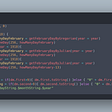# Last Updated: October 16, 2020

Welcome to the fourth episode of Algorithm Spotlight! The featured algorithm for this week is 🥁…drum roll, please…🥁 — Binary Search!

# Preface

We are playing the number guessing game! I am thinking of a number that is between 0–100. If my number is 23, how can you guess my number quickly and efficiently? Welcome to the world of Binary Search.

# Part 1: The Concept of Searching

One of the most frequent daily tasks is searching through a phone book, a book from a bookshelf, etc. Luckily, in computer science, we have plenty of techniques for searching through items. The first technique is linear search, which runs in O(n) time — that means that we need to see every single element in the array of size `n`. The pseudocode for this is shown below:

It turns out, even though this works, this is impractical in the real world when the input data can get large. Linear search doesn’t work on an array, but what if it was a sorted array? It turns out Binary Search is perfect for this, plus it runs in O(log n) — logarithmic time, which means it divides the search space in half at each step and is WAY FASTER than linear search.

# Part 2: Binary Search 🔎

Binary Search is based on the Divide and Conquer technique. Divide and Conquer is split into three steps:

Divide: Divide the problem into small subproblems.

Conquer: Recursively solve each subproblem.

Combine: Combine all the solved subproblems for the final solution.

Binary Search repeatedly divides the problem into two subproblems. At each step, based on our given constraints, we keep only one subproblem through the fact that the items are sorted. Take a look at the pseudocode of this algorithm:

The Binary Search algorithm works by defining `low` and `high` variables that represent the starting and ending indexes of where the element can possibly be found. Then, as long as the `low` and `high` variables are valid indexes (`low <= high`), we do the following tasks:

1. Calculate the middle element’s index (`low + (high — low) / 2` is preferred over `(low + high) / 2` to prevent overflow). This value is typically named `mid` .
2. Get the element at index `mid` and set this value as `guess`.
3. Check through the following cases:
• If the guess is the target value, then return the index found (`mid` ).
• If the guess is less than the target value, the array from the index `0` to index `low` is “wiped out” as the target element is definitely not present in that interval. To “wipe out” and decrease the search space in half, we set `low = mid + 1` for the next iteration’s middle element index calculation.
• If the guess is greater than the target value, the array from `high` to the end of the array is “wiped out.” We then change `high` to `high = mid — 1` .

If the condition `low <= high` fails, then we know for that `target` is not in the array, so we return an error code (normally `-1`).

To help you understand Binary Search better, here’s an awesome visualization of this in action:

Now that you understand Binary Search let’s move on to some limitations of Binary Search!

# Part 3: Limitations of Binary Search

Binary Search is awesome, except there are a few limitations that outweigh the speed of Binary Search:

1. The array must be sorted. Most programming languages have quick sorting algorithms that run in O(n log n) time. Even before Binary Search, the time complexity is even worse than linear time, which runs in O(n)!
2. Binary Search only works on data structures that are indexed (e.g., array) or have direct access to elements. If we can’t directly access elements in data structures, we cannot apply Binary Search to it.

# Part 4: Find First & Last Position of Element in a Sorted Array

Clearly, based on the title “…Sorted Array”, we can see Binary Search is appropriate for this problem. For this problem, we will be using two modified versions of Binary Search:

• `upper_bound(arr, x)` — the index of the first item in the array `arr`that is greater than target `x`
• `lower_bound(arr, x)` — the index of the first item in the array `arr` that is equal to target `x`

Now that we know `upper_bound` and `lower_bound` , this problem is super easy to solve. The first position is `lower_bound` and the last position is `upper_bound — 1` , as we need to return the last position of the element `x`. If the result of `lower_bound` is greater than `upper_bound` (which is impossible as the start value cannot be greater than the ending value), we can return `[-1, -1]` . Refer to the pseudocode below that implements this quite elegantly:

# Part 5: Practice Problems

As practice makes perfect, here are some Binary Search problems to test your skills to some fun problems:

Hints:

Hints:

1. This is just the Binary Search problem in disguise.
2. Implement Binary Search, except change the conditional statements to match the guessing game.

# Thanks for reading and don’t miss out on the next episode of Algorithm Spotlight!

--

--

--

## More from Aaron Ma

Living in The Milky Way 😀

Love podcasts or audiobooks? Learn on the go with our new app.

## Converting Numerical variable to Categorical in Python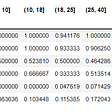## Build My First App(VII)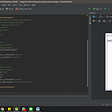## I Sell Triangles on the Internet## Teaching Girls to Code at U Girls Code — Year 2## Axure Tutorial: Left Navigation Adapt to the Height of the Browser Window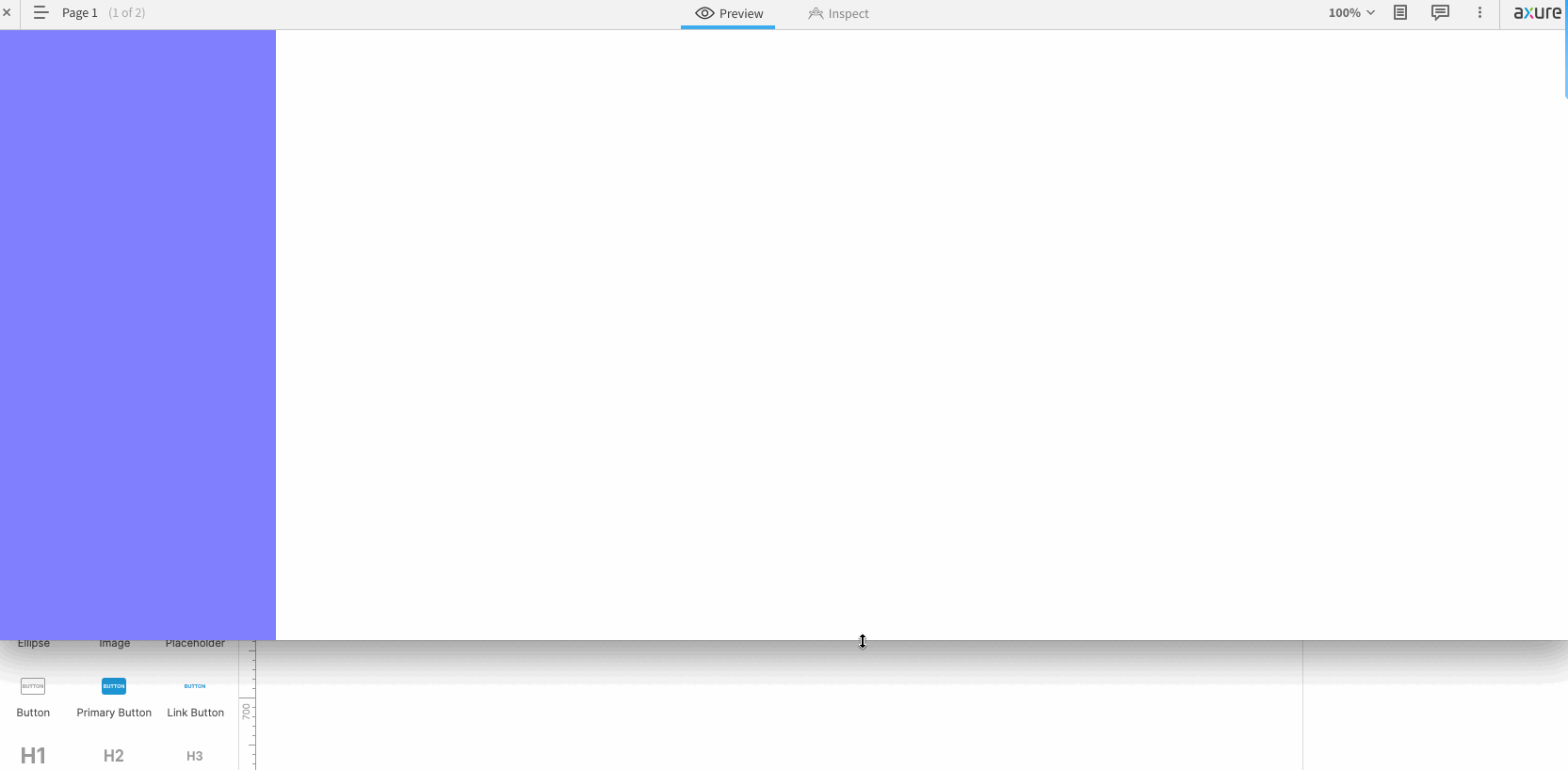## Satisfying a quirk of mine.## Aaron Ma

Living in The Milky Way 😀

## Monitoring Certificate Expiry Dates of a JAVA Keystore (.JKS)## How to solve the “Fibonacci Number modulo M” Problem in Java## Implement PageRank Algorithm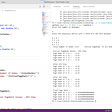## Hackerrank: Day Of The Programmer